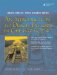# Review Questions

1.

What is a stream? What kinds of streams are there?

2.

Give one reason to use an ostrstream.

3.

What is the main difference between getline and the >> operator?

4.

What is the type of each expression?

1. 3.14
2. 'D'
3. "d"
4. 6
5. 6.2f
6. "something stringey"
7. false
5.

In Example 1.23, identify the type and value of each "thing":

Example 1.23. src/types/types.cpp

 ```#include int main() { using namespace std; int i = 5; int j=6; int *p = &i; <-- 1 int& r=i; int& rpr=(*p); i = 10; p = &j; <-- 2 rpr = 7; <-- 3 r = 8; <-- 4 cout << "i=" << i << " j=" << j << endl; <-- 5 } ```   (1)*p: ______ (2)*p: ________ (3)*p: ________ (4)rpr: ________ (5)i: ________ j: ________
6.

What is the difference between a pointer and a reference?

7.

Why does main(int argc, char* argv[]) sometimes have parameters? What are they used for?An Introduction to Design Patterns in C++ with Qt 4
ISBN: 0131879057
EAN: 2147483647
Year: 2004
Pages: 268